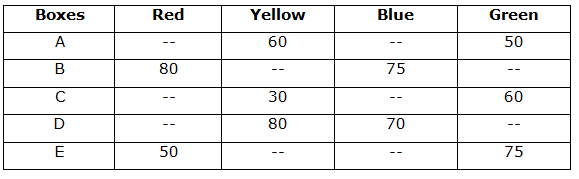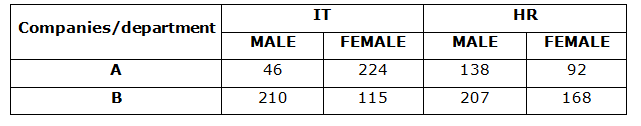# “The Knowledge Library”

Knowledge for All, without Barriers……….

# Data Interpretation-9

Direction (1 – 5): Study the following information carefully and answer the given questions?

The given table shows the number of non-defective balls in four different colors of 5 different boxes. (Some of the data is missing).1) If the total number of non-defective balls in box B in all the colors together is 300 and the number of non-defective yellow balls in B is 20% more than the number of nondefective blue balls in B, then what is the average number of non-defective green balls in A, B and C together?

a) 50

b) 60

c) 45

d) 40

e) 55

2) The total number of defective balls in C is 200. If the average number of non defective yellow, blue and green balls in box C is 45 and the ratio of the total number of defective balls to total number of non defective balls in C is 8:7, what is the number of non defective red balls in C?

a) 20

b) 40

c) 30

d) 10

e) 25

3) The ratio of the number of non defective yellow balls in E to the number of non defective Blue balls in E is 8: 7 and the total number of non defective balls in E is 275. The number of non defective green balls in C is approximately what percent of the number of non defective yellow balls in E?

a) 60%

b) 65%

c) 70%

d) 75%

e) 80%

4) Total number of non defective balls in D is 315 and the ratio of the number of non defective red balls in D to the number of non defective green balls in D is 5: 6. What is the number of defective green balls in D if the ratio number of non defective to defective green balls in D is 9: 10?

a) 80

b) 50

c) 60

d) 70

e) None of these

5) If the number of non defective yellow balls in B is 50% more than the number of non defective yellow balls in A and the number of non defective yellow balls in A is 25% less than the number of non defective yellow balls in E, then what is the average number of non defective yellow balls in all the 5 boxes together?

a) 63

c) 64

c) 66

d) 68

e) None of these

Caselet

Direction (6 – 10): Read the following information carefully and answer the given questions?

There are 1200 employees in two different companies A and B and each company have two different departments – IT and HR. Total number of employees in company A is 500. 115 IT department employees from company B is female. The number of female employees from company B in HR department is 25% less than the number of female employees from A in IT department and 30 more than number of male employees from company A in HR department. Ratio of the number of male employees from company A in IT department to the number of female employees from A in HR department is 1: 2. Ratio of the number of male employees from B in IT department to the number of female employees from B in HR department is 5: 4. The number of male employees from B in HR department is 207.

6) If the salary of each female person in the IT department from company A is Rs. 2000 more than that of salary of each male person from same company at same department, And the total salary of all the person in the IT department from company A is Rs. 3148000, then what is the salary of each male and female person of IT department of company A respectively?

a) Rs.12000, Rs.10000

b) Rs.13000, Rs.15000

c) Rs.16000, Rs.18000

d) Rs.10000, Rs.12000

e) Rs.15000, Rs.13000

7) What is the ratio of male person from HR department in company A to the male person from IT department in company B?

a) 26: 47

b) 23: 45

c) 23: 35

d) 47: 98

e) None of these

8) What is the average of total number of male person from company A and B?

a) 300.50

b) 275.75

c) 325.25

d) 400.25

e) 335.50

9) If 50% of Female people only from HR department on both the companies are went for team lunch, what is the difference between all the male person in the IT department and the persons went for team lunch?

a) 116

b) 126

c) 135

d) 156

e) 188

10) If 66.66% of Male person from HR department are getting salary hike on 2020, then what is the sum of female person from both the department and the person who got salary hike in HR department?

a) 729

b) 839

c) 779

d) 629

e) 829

Directions (1-5) :

Number of yellow balls in B = 75 * 120/100 = 90

Number of green balls in B = 300 – 80 – 90 – 75 = 55

Average of the green balls in A, B and C = (50 + 55 + 60) / 3 = 55

Total number of non defective yellow, blue and green balls in C = 45 * 3 = 135

Number of non defective blue balls in C = 135 – 30 – 60 = 45

Total number of non defective balls in C = 7/8 * 200 = 175

Number of non defective red balls in C = 175 – 45 – 30 – 60 = 40

Number of non defective yellow balls in E = 8x

Number of non defective blue balls in E = 7x

8x + 7x + 50 + 75 = 275

15x = 150

x = 10

Number of non defective yellow balls in E = 8 * 10 = 80

Required percentage = 60/80 * 100 = 75%

5x + 6x + 80 + 70 = 315

11x = 165

x = 15

Number of non defective green balls = 6 * 15 = 90

Number of defective green balls = 10/9 * 90 = 100

Number of non defective yellow balls in B =150/100 * 60 = 90

Number of non defective yellow balls in E = 100/75 * 60 = 80

Average = (60 + 90 + 80 + 30 + 80)/5 = 68

Directions (6 – 10):

Number of female employee from A in IT department = x

Number of female employees from B in HR department = x * 75/100 = 3x/4

Number of male employees from A in HR department = 3x/4 – 30

Number of male employees from B in IT department = 5/4 * 3x/4 = 15x/16

Total number of employees = 1200,

Total number of employee in Company A = 500

Total number of employee in Company B

= Total number of employees – Total number of employee in Company A

= 1200 – 500 = 700

Number of female employees from company B from IT department = 115

The number of male employees from B in HR department = 207

15x/16 + 115 + 207 + 3x/4 = 700

27x/16 = 700 – 322

27x/16 = 378

x = 224

Number of female employee from A in IT department = x = 224

Number of female employees from B in HR department =3x/4 = 168

Number of male employees from A in HR department = 3x/4 – 30 = 138

Number of male employees from B in IT department = 15x/16 = 210

Ratio of the number of male employees from company A in IT department (P) to the number of female employees from A in HR department (Q)

= P/Q = 1: 2 –> (1)

Total number of employee in Company A = 500

P + 224 + 138 + Q = 500

P + Q = 500 – 362

P + Q = 138 –> (2)

On solving (1) and (2) => P = 46, Q = 9646 * (X) + 224 * (X + 2000) = 3148000

X = 10000

X = 10000 – Each Male person salary

X + 2000 = 12000 = Each Female person salary

Ratio of male person from HR department in company A to the male person from IT department in company B

= > 138 / 210 = 23: 35

Average = (46 + 210 + 138 + 207) / 4 = 601/2 = 300.50

50% of Female people only from HR department on both the companies are went for team lunch = 50% of (92 + 168)

= 50% of 260
= 130

Difference = 256 – 130 = 126

66.66% of Male person from HR department

= 2/3 of 345

= 230

Female person from both the department = 599

Sum = 599 + 230 = 829